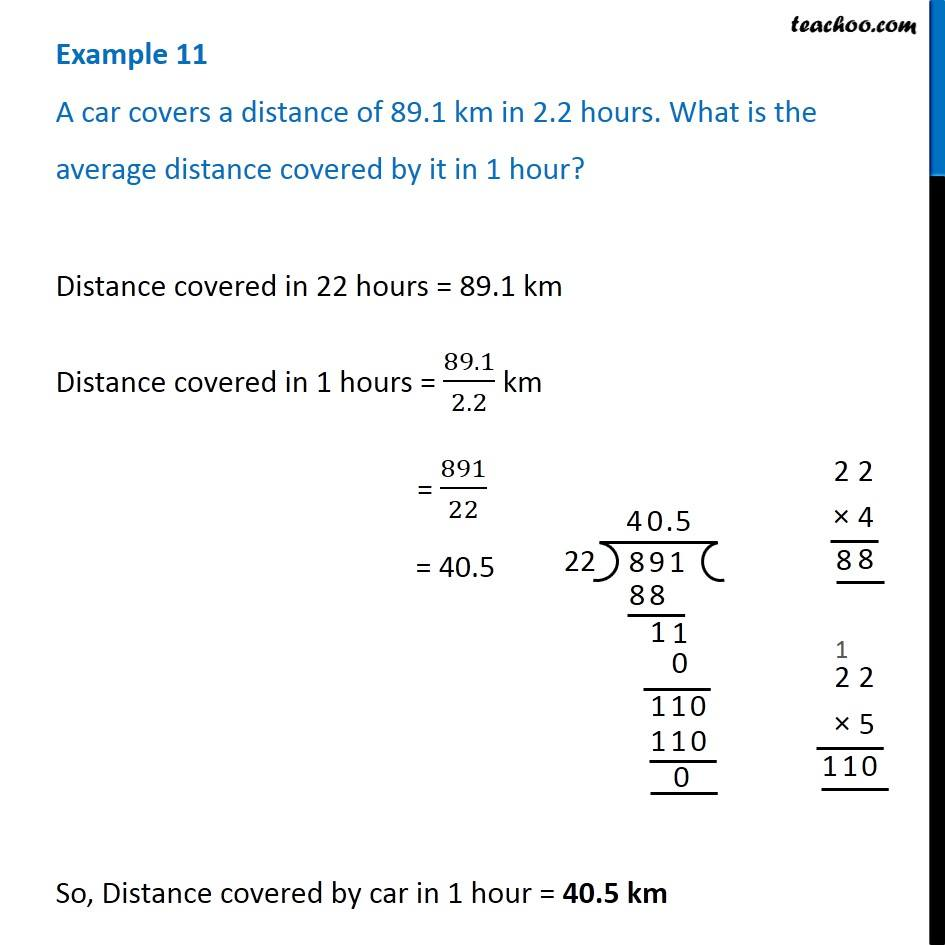1. Chapter 2 Class 7 Fractions and Decimals
2. Concept wise
3. Division of Decimals - Statement Questions

Transcript

Example 11 A car covers a distance of 89.1 km in 2.2 hours. What is the average distance covered by it in 1 hour?Distance covered in 22 hours = 89.1 km Distance covered in 1 hours = 89.1/2.2 km = 891/22 = 40.5 So, Distance covered by car in 1 hour = 40.5 km

Division of Decimals - Statement Questions